Next: Statics Up: Angular momentum Previous: Worked example 9.2: Angular

## Worked example 9.3: Spinning skater

Question: A skater spins at an initial angular velocity of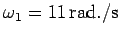with her arms outstretched. The skater then lowers her arms, thereby decreasing her moment of inertia by a factor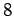. What is the skater's final angular velocity? Assume that any friction between the skater's skates and the ice is negligible.

Answer: Neglecting any friction between the skates and the ice, we expect the skater to spin with constant angular momentum. The skater's initial angular momentum is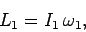where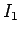is the skater's initial moment of inertia. The skater's final angular momentum is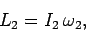where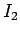is the skater's final moment of inertia, and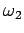is her final angular velocity. Conservation of angular momentum yields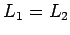, or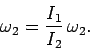Now, we are told that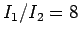. Hence,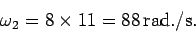Richard Fitzpatrick 2006-02-02